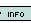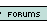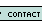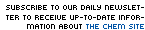1. Hydrochloric acid reacts with calcium carbonate yielding calcium chloride, water, and carbon dioxide. How many moles of calcium carbonate would be necessary to react with 8 moles of HCl? 1 2 3 4 5 2. Balance the following equation (find what number coefficient X is): NH3 (g) + O2 (g) --> NO (g) + XH2O (g). 2 3 4 5 6 3. What is the mass percent of oxygen in H2SO4 (sulfuric acid)? 0 1% 2.04% 32.70% 65.26% 4. On a faraway planet, the following system of measurement is used: 1 zorg = 5.81 zaks 1 zak = .87 zips 1 zip = 2.52 zeeks 1 zeek = 10.02 yaks 1 yak = 3.91 zaps How many zorgs are in 3.20 zaps? 5.81 12.53 2.74 0.00641 None of these 5. When 2 moles of propane (C3H8, MW = 44 grams) is combusted with oxygen gas, how many grams of CO2 (MW: 44 grams) are produced? 88 44 3 132 264 6. A chemistry student was performing an experiment involving the following reaction: C3H8 (g) + O2 (g) --> CO2 (g) + H2O (g), balancing it with the coefficients 1, 5, 2, and 4 respectively. The oxygen was in excess. He used 20.18 grams of C3H8. When he measured the amount of CO2 produced, he was astounded to find that 46.22 grams were produced, 5.88 grams more than the theoretical yield of 40.34. What went wrong? Hint: Look at the formula C3H8's coefficient should be 3, not 1 CO2's coefficient should be 3, not 2 O2's coefficient should be 3, not 5 Water's coefficient should be 3, not 4 None of these 7. The formation of ammonia is shown by the following reaction: N2 (g) + 3H2 (g)--> 2NH3 (g). You are given 13.85 grams of nitrogen and 5.27 grams of hydrogen. If the experiment produced 3.297 grams of ammonia, then what is the percent yield? 25% 50% 35.80% 76.60% None of these 8. When aluminum reacts with sulfuric acid, the result is aluminum sulfate and hydrogen gas. How many moles of sulfuric acid (H2SO4) will react with 24 moles of aluminum? 12 14 16 18 20 9. How many grams is 0.25 moles of table salt (NaCl?) 58.44 grams 14.61 grams 14.6 grams 58 grams 15 grams 10. What is the % mass of carbon in carbon dioxide? 44% 25% 27% 63% 50% 11. 6.02x1023 is.... a random number Avogadro's number stoichiometry percent yield None of these 12. How many carbon molecules are present in 5.0 grams of glucose? (C6H12O6) 1.0 * 10^22 5.1 * 10^22 1.0 * 10^23 6 None 13. How many kilometers are in 10.00 miles? (1 inch equals exactly 2.54 centimeters) 2.04 km 4.09 km 8.09 km 16.09 km None of these 14. If 11 grams in a reaction was obtained, and you had a theoretical yield of 14 grams, what is your % yield? 0.79% 79% 11% 14% 12% 15. The empirical formula of a compound is C10H20 with a molar mass of 284 grams, find the molecular weight. C20H40 C10H20 C5H10 C4H8 CH4 Name: E-mail: Last 15 Challengersnews | about us | contact us tutorials index | organic chemistry | practice tests | online quizzes | reference tools site copyright (c) 2002 to neopages.com